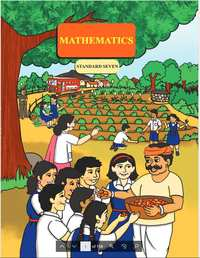# Balbharati solutions for Mathematics 7th Standard Maharashtra State Board chapter 17 - Miscellaneous Problems : Set 2 [Latest edition]

#### Chapters## Chapter 17: Miscellaneous Problems : Set 2

ExerciseMultiple choice Questions
Exercise [Page 100]

### Balbharati solutions for Mathematics 7th Standard Maharashtra State Board Chapter 17 Miscellaneous Problems : Set 2 Exercise [Page 100]

Exercise | Q 1 | Page 100

Angela deposited 15000 rupees in a bank at a rate of 9 p.c.p.a. She got simple interest amounting to 5400 rupees. For how many years had she deposited the amount?

Exercise | Q 2 | Page 100

Ten men take 4 days to complete the task of tarring a road. How many days would 8 men take?

Exercise | Q 3 | Page 100

Nasruddin and Mahesh invested Rs 40,000 and Rs 60,000 respectively to start a business. They made a profit of 30%. How much profit did each of them make?

Exercise | Q 4 | Page 100

The diameter of a circle is 5.6 cm. Find its circumference.

Exercise | Q 5.1 | Page 100

Expand: (2a – 3b)2

Exercise | Q 5.2 | Page 100

Expand: (10 + y)2

Exercise | Q 5.3 | Page 100

Expand: ("p"/3 + "q"/4)^2

Exercise | Q 5.4 | Page 100

Expand: ("y" - 3/"y")^2

Exercise | Q 6.1 | Page 100

Use a formula to multiply of (x - 5)(x + 5).

Exercise | Q 6.2 | Page 100

Use a formula to multiply of (2a – 13)(2a + 13)

Exercise | Q 6.3 | Page 100

Use a formula to multiply of (4z – 5y)(4z + 5y)

Exercise | Q 6.4 | Page 100

Use a formula to multiply of (2t – 5)(2t + 5)

Exercise | Q 7 | Page 100

The diameter of the wheel of a cart is 1.05 m. How much distance will the cart cover in 1000 rotations of the wheel?

Exercise | Q 8 | Page 100

The area of a rectangular garden of length 40 m, is 1000 sqm. Find the breadth of the garden and its perimeter. The garden is to be enclosed by 3 rounds of fencing, leaving an entrance of 4 m. Find the cost of fencing the garden at a rate of 250 rupees per metre.

Exercise | Q 9 | Page 100

From the given figure, find the length of hypotenuse AC and the perimeter of ∆ABC.

Exercise | Q 10 | Page 100

If the edge of a cube is 8 cm long, find its total surface area.

Exercise | Q 11 | Page 100

Factorise:  365y4z3 – 146y2z

Multiple choice Questions [Page 100]

### Balbharati solutions for Mathematics 7th Standard Maharashtra State Board Chapter 17 Miscellaneous Problems : Set 2 Multiple choice Questions [Page 100]

Multiple choice Questions | Q 1 | Page 100

Choose the right answers from the option:

If the average of the numbers 33, 34, 35, x , 37, 38, 39 is 36, what is the value of x?

• 40

• 32

• 42

• 36

Multiple choice Questions | Q 2 | Page 100

Choose the right answers from the option:

The difference of the squares, (612 – 512 ) is equal to ______.

• 1120

• 1230

• 1240

• 1250

Multiple choice Questions | Q 3 | Page 100

Choose the right answers from the option.

If 2600 rupees are divided between Sameer and Smita in the proportion 8 : 5, the share of each is _______ and _______ respectively.

• Rs 1500, Rs 1100

• Rs 1300, Rs 900

• Rs 800, Rs 500

• Rs 1600, Rs 1000

## Chapter 17: Miscellaneous Problems : Set 2

ExerciseMultiple choice Questions## Balbharati solutions for Mathematics 7th Standard Maharashtra State Board chapter 17 - Miscellaneous Problems : Set 2

Balbharati solutions for Mathematics 7th Standard Maharashtra State Board chapter 17 (Miscellaneous Problems : Set 2) include all questions with solution and detail explanation. This will clear students doubts about any question and improve application skills while preparing for board exams. The detailed, step-by-step solutions will help you understand the concepts better and clear your confusions, if any. Shaalaa.com has the Maharashtra State Board Mathematics 7th Standard Maharashtra State Board solutions in a manner that help students grasp basic concepts better and faster.

Further, we at Shaalaa.com provide such solutions so that students can prepare for written exams. Balbharati textbook solutions can be a core help for self-study and acts as a perfect self-help guidance for students.

Concepts covered in Mathematics 7th Standard Maharashtra State Board chapter 17 Miscellaneous Problems : Set 2 are Concept of Direct Proportion, Concept of Inverse Proportion, Partnership, Variations, Bank, Concept of Principal, Interest, Amount, and Simple Interest, Arc of the Circle, Circumference of a Circle, Concept of Circle - Centre, Radius, Diameter, Arc, Sector, Chord, Segment, Semicircle, Circumference, Interior and Exterior, Concentric Circles, Central Angle and the Measure of an Arc, Area of Rectangle, Area of a Triangle, Surface Area of a Cuboid, Perimeter of Polygon, Concept of Perimeter, Concept of Area, Area of Square, Surface Area of a Cube, Perimeter of a Rectangle, Right-angled Triangles and Pythagoras Property, Expansion of (a + b)2 = a2 + 2ab + b2, Factorising Binomial, Expansion of (a - b)2 = a2 - 2ab + b2, Expansion of (a + b)(a - b), Factorising Algebraic Expressions, Frequency Distribution Table, Concept of Representative Values - Average, Concepts of Statistics.

Using Balbharati 7th Standard solutions Miscellaneous Problems : Set 2 exercise by students are an easy way to prepare for the exams, as they involve solutions arranged chapter-wise also page wise. The questions involved in Balbharati Solutions are important questions that can be asked in the final exam. Maximum students of Maharashtra State Board 7th Standard prefer Balbharati Textbook Solutions to score more in exam.

Get the free view of chapter 17 Miscellaneous Problems : Set 2 7th Standard extra questions for Mathematics 7th Standard Maharashtra State Board and can use Shaalaa.com to keep it handy for your exam preparation